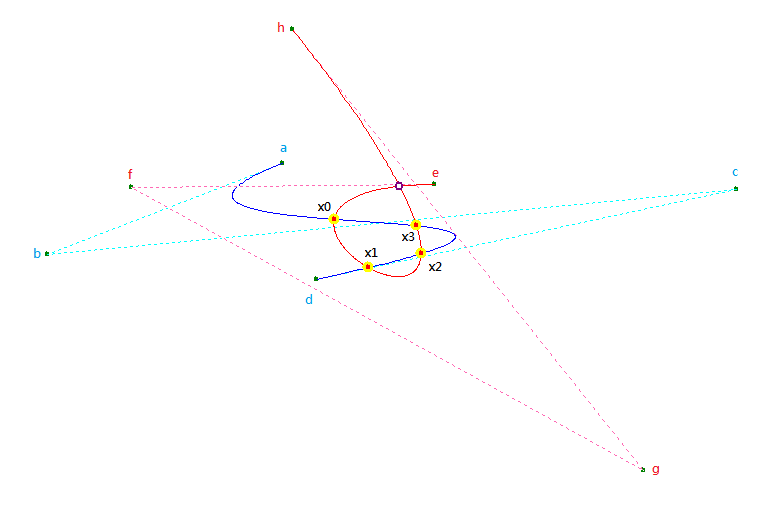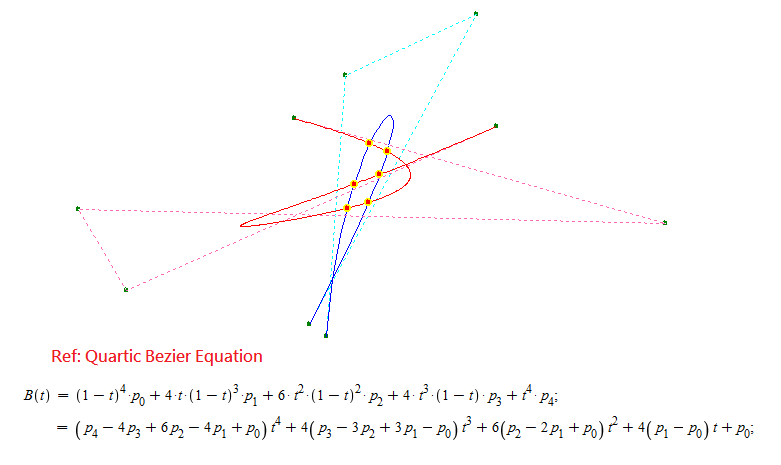Intel® oneAPI Math Kernel Library
Ask questions and share information with other developers who use Intel® Math Kernel Library.
Announcements
This community is designed for sharing of public information. Please do not share Intel or third-party confidential information here.
6590 Discussions

## Fast and precise INTERSECTION solution of cubic BEZIER curvesBeginner
422 Views

To whom it may concern,

Cubic Bezier curve has been invented over fifty years, people are all expecting its great functionality and productivity to be used in CAD/CAM for free form curve design or photo-realistic solid modeling. However I believeits usage was limited as there is no effective way to solve the intersection points problem either in between curve-to-curve or within a curve itself.

Anyway, the problem is a history now. Enclosed are a picture of cubic Bezier curves intersection points and certain data related with the two curves that auto-generated by a program. Anyone, who feels interested are all welcome to verify them for the truth.

Regards,

Hunt ChangBlue Curve control points: P0 (404.000000, 355.000000), P1 (169.000000, 264.000000), P2 (858.000000, 329.000000), P3 (438.000000, 239.000000);

Red Curve control points: P0 (556.000000, 334.000000), P1 (253.000000, 331.000000), P2 (765.000000, 48.000000), P3 (414.000000, 489.000000);
Self Intersection point at (521.381253777, 332.148294653); t = 0.844680894, t = 0.042885836;

The Intersection point(s) of those two curves:

x0(455.734152990, 299.109787062), x1(490.237592592, 250.732158097), x2(542.630905524, 264.568535791), x3(538.123536369, 292.686435169);

Blue Curve's t value(s):

t = 0.437206056, t = 0.952837683, t = 0.883884689, t = 0.596112430;

Red Curve's t value(s):

t = 0.232537221, t = 0.453969337, t = 0.713303391, t = 0.780607425;Ps, my solution is fast, precise and by theory. It is not an approximation approach like bi-section or Bez-clipping or ...
4 RepliesBeginner
422 Views

To: R&D experts of Intel,

Not to boast myself, but if I do not speak for my own solution then nobody can.

People asked me about what is the better of my solution than the other solutions that already exists?

First off, I guess my solution so far is the only one in the whole-world which solved the intersection points problem of cubic and quadratic Bezier curves accurately by Geometry theory instead of by way of approximation. Frankly the way of approximation has nothing wrong as soon as it can get the correct answer; but compare to the same level of data accuracy or precision, I believe mine is much faster. Although my way to solve the problem is also a complicate process, but it is still fast enough to be operated in real-time even by an AMD Athlon(K5) CPU. May be someone would defy me for bluffing, please take a few minutes of calculation to verify the data I already presented here, just let those data speaking for the truth.

Secondly, cause in each intersection point there must be two t values (or more by coincidence) get involved, so we can get (at least) two sets answers of coordinates from each intersection. In theory, when an intersection exists, the two sets of coordinate must be exactly the same, but in reality of computation there are certain deviation exists most of the time. Under a reasonable coordinate setting, my data deviation is always lower than the constant of FLT_EPSILON; actually I had done some random control points location tests, over 97% of my data deviation are lower than 1.0e-10 and only less than 0.3% deviation are within the range of FLT_EPSILON ~ 1.0e-08. Since my solution is by Geometry theory, it means if I change the code to use 16-byte-doulbe-precision format number in the future then the data precision would be double as well; and its extra CPU overhead would be quite small.

Lastly, during the very long period I spent to discover this solution, I did find some math properties of the curve that never have been exposed before; actually I count on them to solve the 2D curve intersection puzzle. So, even I do not know what kind of algorithm or strategy you are using to solve the B-spline-surface intersection problem now, but I think my solution or my discovery may offer you a better chance or a different approach to look at that problem.

If you are interested on my solution, feel free to contact me.

Hunt ChangBeginner
422 Views

# Quartic X Cubic Bezier Curves

To whom it may feel interested,
Hunt ChangBlue Curve control points:

P0(346.000000, 226.000000), P1(365.000000, 487.000000), P2(496.000000, 548.000000), P3(329.000000, 238.000000);

Red Curve control points:

P0(314.000000, 444.000000), P1(685.000000, 339.000000), P2(98.000000, 353.000000), P3(146.000000, 272.000000), P4(516.000000, 436.000000);

The Intersection point(s) of those two curves:

(389.369694260, 418.874980154), (406.963141873, 410.637760315), (388.486672038, 360.281964254), (366.755538541, 354.242638725);

(399.378757587, 387.966095862), (373.797923544, 378.353180514);

Blue Curve's t value(s):

t = 0.348859330, t = 0.731874858, t = 0.837820609, t = 0.194321812;

t = 0.785493970, t = 0.242928969;

Red Curve's t value(s):

t = 0.066832606, t = 0.092449624, t = 0.321249773, t = 0.363412522;

t = 0.910128111, t = 0.885876885;Beginner
422 Views

Thanx for the InfoBeginner
422 Views

How this is included in business section